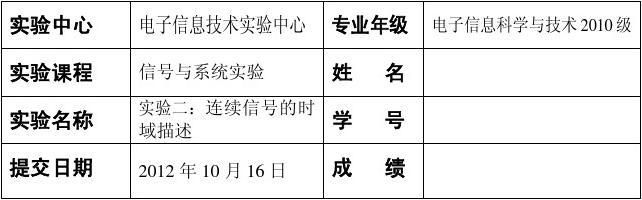• 实验2利用MATLAB分析信号频谱系统频率特性实验2 利用MATLAB分析信号与系统频域特性 实验目的 1.深入理解信号频谱概念，掌握典型信号频谱以及Fourier 变换主要性质及其matlab实现； 2.学习掌握连续时间...
实验2利用MATLAB分析信号频谱和系统的频率特性实验2   利用MATLAB分析信号与系统的频域特性  实验目的 1.深入理解信号频谱的概念，掌握典型信号的频谱以及Fourier 变换的主要性质及其matlab实现； 2.学习和掌握连续时间系统的频率特性及其幅度特性、相位特性的物理意义及其matlab实现；  3.掌握 抽样定理 实验原理一 傅立叶变换和反变换的Matlab实现 Matlab提供了能直接求解傅立叶变换和反变换的函数fourier()、ifourier()。 调用格式分别为：                    F=fourier(f)                    f=ifourier(F)  举例1 syms t x=exp(-2*abs(t)) F=fourier(x) subplot(211) ezplot(x) subplot(212) ezplot(F)  仿真波形 举例2   傅里叶变换的对称性 命令代码1：     syms t     r=0.01;%采样间隔     j=sqrt(-1);     t=-15:r:15;     f=sin(t)./t;%计算采样函数的离散采样点     f1=pi*(Heaviside(t+1)-Heaviside(t-1));%计算脉宽为2的门信号的离散采样点     N=500;%采样点数     k = -N:N;     W=5*pi*1;%设定采样角频率     w=k*W/N;%对频率采样 续  F=r*sinc(t/pi)*exp(-j*t'*w);%计算采样函数的频谱     F1=r*f1*exp(-j*t‘*w);%计算门函数的频谱   subplot(221);plot(t,f);     xlabel('t'); ylabel('f(t)');     subplot(222); plot(w,F);     axis([-2 2 -1 4]);     xlabel('w'); ylabel('F(w)');     subplot(223); plot(t,f1);     axis([-2 2 -1 4]);     xlabel('t'); ylabel('f1(t)');     subplot(224); plot(w,F1);     axis([-20 20 -3 7]);     xlabel('w'); ylabel('F1(w)'); 仿真波形 仿真波形 实验原理二 连续时间系统频率响应的MATLAB实现       系统频率响应，是指系统在正弦信号激励下稳态响应随频率变化的情况，包括幅度随频率的响应和相位随频率的响应两个方面。        Matlab提供了专门对连续时间系统频率响应H(jω)进行分析的函数freqs()。该函数可以求出系统频率响应的数值解，并可绘出系统的幅频和相频响应曲线。 一般调用格式：                        [h,w]=freqs(b,a,n)        其中h为返回w所定义的频率点w上系统频率响应的幅值；b为系统频率响应分子多项式系数，a为系统频率响应分母多项式系数，n为输出频率点个数。 举例3 已知一RLC二阶低通滤波器，该电路的频率响应为      用freqs函数绘出该频率响应。  命令代码： b=[0 0 1];     a=[0.08 0.4 1];     [h,w]=freqs(b,a,100);     h1=abs(h);     h2=angle(h);     subplot(211);     plot(w,h1);     grid     xlabel('角频率(W)');     ylabel('幅度');     title('H(jw)的幅频特性');     subplot(212);     plot(w,h2*180/pi);     grid     xlabel('角频率(W)');     ylabel('相位(度)');     title('H(jw)的相频特性'); 仿真波形 实验原理三   抽样定理的Matlab实现 举例4 用有限时宽余弦信号f(t)=cos(2πt/3)(0≤t ≤40)近似理想余弦信号，用Matlab编程画出该信号及其抽样信号的频谱，并对比观察过抽样和欠抽样状态。 解：首先计算该信号的临界抽样角频率 临界抽样频率 临界抽样周期 * * x =   exp(-2*abs(t))     F =   4/(4+w^2) 时域抽样定理 一个频谱受限的信号           ， 如果频谱只占据                          的范围， 则信号          可以用等间隔的抽样值唯一的表示。而抽样间隔必须
展开全文• 利用MATLAB绘制二阶控制系统单位阶跃响应曲线 作者张宇涛 张怀超 陈佳伟 一课设目的和意义 学习控制系统单位阶跃响应 记录单位阶跃响应曲线 比较阻尼比zeta为不同值时曲线变化趋势 掌握二阶系统时间响应分析...
• 2 2 利用 MATLAB 绘制二阶控制系统单位阶跃响应曲线 作者张宇涛 张怀超 陈佳伟 一课设目的和意义 学习控制系统单位阶跃响应 记录单位阶跃响应曲线 比较阻尼比 zeta 为不同值时曲线变化趋势 掌握二阶系统时间...
• 毕业设计 毕业论文题目 基于 matlab 的指纹识别 院 系 班 级 学生姓名 号 指导教师 时间 目录 摘要 4 Abstract 4 第一章 绪论5 1.1 研究背景 5 1.2 国内外研究现状 6 1.3 研究的目的和意义 8 1.4 研究内容 9 第二...
• 毕业设计开题报告理工类 设计题目 基于MATLAB的数字滤波器设计 学生姓名 学号 专业 电子信息工程 一 课题的目的意义 这次毕业设计的主要目的是进一步学习巩固我们在大学这几年所的知识尤其是数字信号处理中的...
• 利用MATLAB绘制二阶控制系统单位阶跃响应曲线 作者张宇涛 张怀超 陈佳伟 一课设目的和意义 学习控制系统单位阶跃响应 记录单位阶跃响应曲线 比较阻尼比zeta为不同值时曲线变化趋势 掌握二阶系统时间响应分析...
• 实验报告一、实验目的1、学习MATLAB 基本用法。2、熟悉常见连续信号的意义、特性及波形。3、学会使用MATLAB 表示信号方法并绘制信号波形。4、观察并熟悉这些信号波形特性。二、实验设备计算机，Matlab 软件...
实验报告一、实验目的1、学习MATLAB 的基本用法。2、熟悉常见连续信号的意义、特性及波形。3、学会使用MATLAB 表示信号的方法并绘制信号波形。4、观察并熟悉这些信号的波形和特性。二、实验设备计算机，Matlab 软件三、实验原理信号一般是随时间而变化的某些物理量。按照自变量的取值是否连续，信号分为连续时间信号和离散时间信号，一般用()f t 和()f k 来表示。若对信号进行时域分析，就需要绘制其波形，如果信号比较复杂，则手工绘制波形就变得很困难，且难以精确。MATLAB 强大的图形处理功能及符号运算功能，为实现信号的可视化及其时域分析提供了强有力的工具。根据MATLAB 的数值计算功能和符号运算功能，在采用适当的MATLAB 语句表示出信号后，就可以利用MATLAB 中的绘图命令绘制出直观的信号波形了。下面分别介绍连续时间信号和离散时间信号的MATLAB 表示及其波形绘制方法。(1)指数信号atAe指数信号atAe在MATLAB 中可用exp 函数表示，其调用形式为： y=A*exp(a*t)(2)正弦信号正弦信号Acos(0ω*t+?)和Asin(0ω+?)分别用MATLAB 的内部函数cos 和sin 表示，其调用形式为：A*cos(0ω*t+phi)和 A*sin(0ω*t+phi)(3)抽样函数Sa(t)在MA TLAB 中用sinc 函数表示，其定义为：Sinc(t)=sin(πt)/(πt)其调用形式为： Y=sinc(t) (4)矩形函数矩形脉冲信号在MA TLAB 中用rectpuls 函数来表示，其调用形式为： y=rectpuls(t,width)
展开全文• 苏州市职业大学 毕业设计开题报告 学院部 专业班级 姓名 号 题目 基于matlab的通信系统仿真 一选题的目的和意义 Matlab是集数值计算图形绘制图像处理系统仿真等强大功能于一体的科学计算语言 将MATLAB图形绘制和...
• 实验利用MATLAB分析信号频谱及系统频率特性实验...学习掌握连续时间系统频率特性及其幅度特性、相位特性物理意义及其matlab实现； 3.掌握 抽样定理 实验原理一 傅立叶变换反变换的Matlab实现 Matlab提供了...
实验利用MATLAB分析信号频谱及系统的频率特性实验2   利用MATLAB分析信号与系统的频域特性  实验目的 1.深入理解信号频谱的概念，掌握典型信号的频谱以及Fourier 变换的主要性质及其matlab实现； 2.学习和掌握连续时间系统的频率特性及其幅度特性、相位特性的物理意义及其matlab实现；  3.掌握 抽样定理 实验原理一 傅立叶变换和反变换的Matlab实现 Matlab提供了能直接求解傅立叶变换和反变换的函数fourier()、ifourier()。 调用格式分别为：                    F=fourier(f)                    f=ifourier(F)  举例1 syms t x=exp(-2*abs(t)) F=fourier(x) subplot(211) ezplot(x) subplot(212) ezplot(F)  仿真波形 举例2   傅里叶变换的对称性 命令代码1：     syms t     r=0.01;%采样间隔     j=sqrt(-1);     t=-15:r:15;     f=sin(t)./t;%计算采样函数的离散采样点     f1=pi*(Heaviside(t+1)-Heaviside(t-1));%计算脉宽为2的门信号的离散采样点     N=500;%采样点数     k = -N:N;     W=5*pi*1;%设定采样角频率     w=k*W/N;%对频率采样 续  F=r*sinc(t/pi)*exp(-j*t'*w);%计算采样函数的频谱     F1=r*f1*exp(-j*t‘*w);%计算门函数的频谱   subplot(221);plot(t,f);     xlabel('t'); ylabel('f(t)');     subplot(222); plot(w,F);     axis([-2 2 -1 4]);     xlabel('w'); ylabel('F(w)');     subplot(223); plot(t,f1);     axis([-2 2 -1 4]);     xlabel('t'); ylabel('f1(t)');     subplot(224); plot(w,F1);     axis([-20 20 -3 7]);     xlabel('w'); ylabel('F1(w)'); 仿真波形 仿真波形 实验原理二 连续时间系统频率响应的MATLAB实现       系统频率响应，是指系统在正弦信号激励下稳态响应随频率变化的情况，包括幅度随频率的响应和相位随频率的响应两个方面。        Matlab提供了专门对连续时间系统频率响应H(jω)进行分析的函数freqs()。该函数可以求出系统频率响应的数值解，并可绘出系统的幅频和相频响应曲线。 一般调用格式：                        [h,w]=freqs(b,a,n)        其中h为返回w所定义的频率点w上系统频率响应的幅值；b为系统频率响应分子多项式系数，a为系统频率响应分母多项式系数，n为输出频率点个数。 举例3 已知一RLC二阶低通滤波器，该电路的频率响应为      用freqs函数绘出该频率响应。  命令代码： b=[0 0 1];     a=[0.08 0.4 1];     [h,w]=freqs(b,a,100);     h1=abs(h);     h2=angle(h);     subplot(211);     plot(w,h1);     grid     xlabel('角频率(W)');     ylabel('幅度');     title('H(jw)的幅频特性');     subplot(212);     plot(w,h2*180/pi);     grid     xlabel('角频率(W)');     ylabel('相位(度)');     title('H(jw)的相频特性'); 仿真波形 实验原理三   抽样定理的Matlab实现 举例4 用有限时宽余弦信号f(t)=cos(2πt/3)(0≤t ≤40)近似理想余弦信号，用Matlab编程画出该信号及其抽样信号的频谱，并对比观察过抽样和欠抽样状态。 解：首先计算该信号的临界抽样角频率 临界抽样频率 临界抽样周期 * * x =   exp(-2*abs(t))     F =   4/(4+w^2) 时域抽样定理 一个频谱受限的信号           ， 如果频谱只占据                          的范围， 则信号          可以用等间隔的抽样值唯一的表示。而抽样间隔必须
展开全文• 设计题目基于MATLAB的电炉温度控制算法比较及仿真研究 系 别 班 级 号 姓 名 指 导 教 师 任务书 一设计的目的意义 掌握所课程的知识综合应用充分认识理论知识对应用技术的指导性作用进一步加强理论知识与...
• 设计题目基于MATLAB的电炉温度控制算法比较及仿真研究 系 别 班 级 号 姓 名 指 导 教 师 任务书 一设计的目的意义 掌握所课程的知识综合应用充分认识理论知识对应用技术的指导性作用进一步加强理论知识与...
• 实验报告一、实验目的1、学习MATLAB的基本用法。2、熟悉常见连续信号的意义、特性及波形。3、学会使用MATLAB表示信号的方法并绘制信号波形。 4、观察并熟悉这些信号的波形特性。二、实验设备计算机，Matlab软件三...实验报告一、实验目的1、学习MATLAB的基本用法。2、熟悉常见连续信号的意义、特性及波形。3、学会使用MATLAB表示信号的方法并绘制信号波形。 4、观察并熟悉这些信号的波形和特性。二、实验设备计算机，Matlab软件三、实验原理信号一般是随时间而变化的某些物理量。按照自变量的取值是否连续，信号分为连续时间信号和离散时间信号，一般用f(t)和f(k)来表示。若对信号进行时域分析，就需要绘制其波形，如果信号比较复杂，则手工绘制波形就变得很困难，且难以精确。MATLAB强大的图形处理功能及符号运算功能，为实现信号的可视化及其时域分析提供了强有力的工具。根据MATLAB的数值计算功能和符号运算功能，在采用适当的MATLAB语句表示出信号后，就可以利用MATLAB中的绘图命令绘制出直观的信号波形了。下面分别介绍连续时间信号和离散时间信号的MATLAB表示及其波形绘制方法。(1)指数信号Ae指数信号Aeatat在MATLAB中可用exp函数表示，其调用形式为： y=A*exp(a*t)(2)正弦信号正弦信号Acos( 0*t+ )和Asin( 0+ )分别用MATLAB的内部函数cos和sin表示，其调用形式为：A*cos( 0*t+phi)和 A*sin( 0*t+phi)(3)抽样函数Sa(t)在MATLAB中用sinc函数表示，其定义为：Sinc(t)=sin( t)/( t)其调用形式为： Y=sinc(t) (4)矩形函数矩形脉冲信号在MATLAB中用rectpuls函数来表示，其调用形式为： y=rectpuls(t,width)
展开全文• 设计题目基于MATLAB的电炉温度控制算法比较及仿真研究 系 别 班 级 号 姓 名 指 导 教 师 任务书 一设计的目的意义 掌握所课程的知识综合应用充分认识理论知识对应用技术的指导性作用进一步加强理论知识与...
• 3、上面只是线性变化，为了达到能处理非线性的目的，u做了个变换，变换的规则传输函数有关 可能还有人问，那么那个阀值是什么呢？简单理解就是让这些数据做了个平移，这就是神经元工作的过程。处理后的结果又...
• 利用MATLAB 绘制二阶控制系统单位阶跃响应曲线作者：张宇涛 张怀超 陈佳伟一：课设目的和意义(1)学习控制系统单位阶跃响应。 (2)记录单位阶跃响应曲线。 (3)比较阻尼比zeta 为不同值时曲线变化趋势。 (4) 掌握...二阶系统响应指标图
• MATLAB的发展历史见下表1-1。(年份不详的请知道的网友补充。) 时间 版本 1985 1.0 1986 2.0（含控制系统工具箱） 1988 3.9 1993 4.0（含Simulink1.0） 1994 4.2(含simulink2.0) 不详 4.3 不详 5.3 2000 6.0...
• 实验一 MATLAB 软件应用复习 一 实验目的意义 熟悉MATLAB软件用户环境 了解MATLAB^件一般目的命令 掌握MATLAB数组操作与运算函数 掌握MATLAB^件基本绘图命令 掌握MATLAB几种循环条件开关选择结构 通过...
• 实用标准文档 实验一 MATLAB软件应用复习 一 实验目的意义 1. 熟悉MATLAB软件用户环境 2. 了解MATLAB软件一般目的命令 3. 掌握MATLAB数组操作与运算函数 4. 掌握MATLAB软件基本绘图命令 5. 掌握MATLAB语言...
• 实用标准文档 实验一 MATLAB 软件应用复习 一 实验目的意义 1. 熟悉 MATLAB 软件用户环境 2. 了解 MATLAB 软件一般目的命令 3. 掌握 MATLAB 数组操作与运算函数 4. 掌握 MATLAB 软件基本绘图命令 5. 掌握 ...
• 为使学生能更好地了解无功补偿装置特性基于 Matlab 电力 电子知识 使学生加深对理论知识理解同时结合仿真验证所知 识 设计来源及背景 ? 随着电力系统中非线性用电设备尤其是电力电子装置应用日 益广泛...
• 河南理工大学物理与电子信息学院 MATLAB基础与应用课程论文 2016 ... 设计原理 1 设计的目的及要求 2 课题的研究意义 2 2. 设计原理 3 采样频率 3 采样位数 3 采样定理 3 时域信号的 FFT分析 3 数字滤波器设计原理
• A 文章编号：论文引用格式：结合区域生长与灰度重建CT图像肺气管树分割彭双，肖昌炎湖南大学电气与信息工程学院, 长沙 410082摘 要：目的：CT图像中肺气管树分割对肺部疾病精确定位与量化评估具有重要意义。...
• 使学生了解掌握利用各种图像采集设备__图像扫描仪、数码照相机、录像机、数码摄像机等获取多种格式静态、动态图像数据方法及手段，了解图像增强、图像分割、图像理解分析算法物理意义；了解图像传输、图像...
• 资学习网 ------------------------------------------------------------精品文档-------------------------------------------------------- 函数实现哈夫曼编码算法编写Matlab 设计目的和意义一 在数字信号...
• 的目的：没什么目的，万一将来用得上呢？！我的希望： 1、有C语言基础和MATLAB基本矩阵计算实践的童鞋们可以无障碍阅读代码； 2、写这些东西的人不是大神，所以不希望大神前来吐槽。仅供包括我在内的菜鸟们交流...智能算法 蚁群算法
• 对图像中物体个数的计算以及车道线检测应用，不仅需要对采集到的图像进行预处理，而且要针对特定的目的进行具体的应用程序开发。为了提高程序的运行效率，使检测结果更具实时性鲁棒性，本课题在Windows 操作系统上...
• 六十年代BagleyRosenberg等先驱在对这些研究成果进行分析与理解基础上，借鉴其相关内容知识，特别是遗传方面理论与概念，并将其成功应用于工程科学某些领域，收到了良好效果。时至八十年代中期，美国...
• 在系统分析设计中，常常把实际系统抽象为一个一般意义系统模型。由于研究目的不同，一个实际系统可以有不同系统模型，一旦获得系统模型，就要建立起系统模型数学方程描述。控制理论主要研究对象是动态...
• 计算机视觉研究的目的是为了让计算机能够利用图像图像序列来识别感知周围的世界,以帮助人们在复杂的情况下解决未知的难题。在智能化信息时代,人类传统的手工劳动逐渐被智能机器人取代,而计算机视觉在此当中占据...
• 本书最新版（第5版）的突出特色是不仅配有MATLAB的应用实例，还在各章章未增加了思考问题来加深读者对该章知识的理解。同时，新版也增加了大量通信系统设计的实例，非常有助于读者理解通信系统的概念及其意义。本书...# 学matlab的目的和意义matlab 订阅